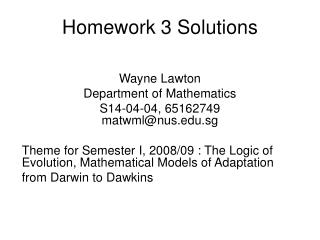# Homework 3 Solutions - PowerPoint PPT PresentationDownload PresentationHomework 3 Solutions

Homework 3 SolutionsDownload Presentation## Homework 3 Solutions

- - - - - - - - - - - - - - - - - - - - - - - - - - - E N D - - - - - - - - - - - - - - - - - - - - - - - - - - -
##### Presentation Transcript

1. Homework 3 Solutions Wayne Lawton Department of Mathematics S14-04-04, 65162749 matwml@nus.edu.sg Theme for Semester I, 2008/09 : The Logic of Evolution, Mathematical Models of Adaptation from Darwin to Dawkins

2. Genotype Ridley page 136 problem 6 Observed Frequency HW Freq. Ratio Fitness Selection Coefficient

3. freq. of in population 1, 2 Ridley page 136 problem 7 migration rate = fraction of population 2 who are first generation immigrants from pop. 1 next generation

4. The effect of assortive mating on a Ridley page 136 problem 8 a locus with 2 alleles A, a with A dominant  2 intermating populations, genotypes freq p(n) of A and q(n) of in gen n in satisfies populations converge to {AA} and {aa}

5. The effect of assortive mating on a Ridley page 136 problem 8 b locus with 2 alleles A, a with 3 phenotypes  3 intermating populations, genotypes Half of the offspring of {Aa} split off each generation so the 3 populations converge to the 2 populations

6. Assortive mating where females mate only with Ridley page 136 problem 8 c i. dominant phenotype fix A (its freq  1) ii. recessive phenotype fix a (its freq  1)

7. Genotype Ridley page 136 problem 9 Generation n Baby Frequency Generation n Adult Frequency (selection against dominant A) Adult Gene Frequency Under the assumption of random mating these generation n adult gene frequencies will equal the generation (n+1) baby gene frequencies

8. We assume that the mutation rate m for a recessive allele a is small and that the selection (with coefficient s) works against the allele a Ridley page 136 problem 10 Let denote the equilibrium frequency for allele a The frequency p(n) in the n-th generation satisfies

9. Assume that for a two allele locus that genotype AA has fitness 1-s, genotype Aa has fitness 1, and genotype aa has fitness 1-t and that random mating occurs. Let p = baby freq. of gene A and q = baby freq. of gene a. Derive formuli for the next baby freq. p’ and q’. Question 11

10. Genotype Answer Question 11 Generation n Baby Frequency Generation n Adult Frequency (selection against homozygytes) Adult Gene Frequencies

11. Assume that in a two allele locus all genotypes have fitness = 1 but that each genotype mates only with the same genotype. Derive equations for the evolution of gene frequencies. Question 12 Remark Same genotype evolution as in problem 8a gene frequencies are constant